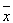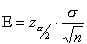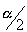# Determining Size of the Sample: Practical Considerations in Sampling and Sample Size

Determining sample size is a very important issue because samples that are too large may waste time, resources and money, while samples that are too small may lead to inaccurate results. In many cases, we can easily determine the minimum sample size needed to estimate a process parameter, such as the population mean.

Sample size determination is the act of choosing the number of observations or replicates to include in a statistical sample. The sample size is an important feature of any empirical study in which the goal is to make inferences about a population from a sample. In practice, the sample size used in a study is determined based on the expense of data collection, and the need to have sufficient statistical power. In complicated studies there may be several different sample sizes involved in the study: for example, in a stratified survey there would be different sample sizes for each stratum. In a census, data are collected on the entire population, hence the sample size is equal to the population size. In experimental design, where a study may be divided into different treatment groups, this may be different sample sizes for each group.

Sample sizes may be chosen in several different ways:

• Experience – A choice of small sample sizes, though sometimes necessary, can result in wide confidence intervals or risks of errors in statistical hypothesis testing.
• Using a target variance for an estimate to be derived from the sample eventually obtained, i.e. if a high precision is required (narrow confidence interval) this translates to a low target variance of the estimator.
• Using a target for the power of a statistical test to be applied once the sample is collected.
• Using a confidence level, i.e. the larger the required confidence level, the larger the sample size (given a constant precision requirement).

When sample data is collected and the sample meanis calculated, that sample mean is typically different from the population mean (µ) . This difference between the sample and population means can be thought of as an error. The margin of error  is the maximum difference between the observed sample mean  and the true value of the population mean (µ) :where:is known as the critical value, the positive Ζ value that is at the vertical boundary for the area ofin the right tail of the standard normal distribution.

σ is the population standard deviation.

n is the sample size.Rearranging this formula, we can solve for the sample size necessary to produce results accurate to a specified confidence and margin of error.This formula can be used when you know  and want to determine the sample size necessary to establish, with a confidence of , the mean value  to within You can still use this formula if you don’t know your population standard deviation  and you have a small sample size. Although it’s unlikely that you know  when the population mean is not known, you may be able to determine  from a similar process or from a pilot test/simulation.

## 2 thoughts on “Determining Size of the Sample: Practical Considerations in Sampling and Sample Size”

error: Content is protected !!